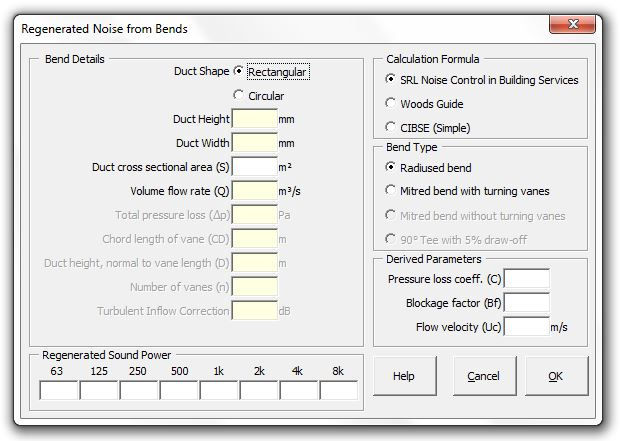### Strutt Help

Regenerated Noise from Bends    1/1

Strutt|Mechanical Services|Regenerated Noise|Bend inserts the sound power level of regenerated noise due to duct bends into the active row of the worksheet.Regenerated noise can be calculated using the Woods, SRL or CIBSE methods.

• Woods calculates regenerated noise from bends using:

• SWL = K + 10logS + 55logV - 45 dB

Where:
SWL is the total sound power level generated by the actual fitting (dB)
K is constant, datum power level of the fitting (given below) (dB)
S is the actual duct area (m2)
V is the actual velocity (m/s)

Values of K (from Table 5.2 Woods):

 90Ḟ Bends K, dB Radiused 48 Mitred with turning vanes 56 Mitred without turning vanes 57 90º tee (5% draw off) 55

Octave band sound power levels are calculated by applying the following corrections (Table 5.2, Woods):

 63 Hz 125 Hz 250 Hz 500 Hz 1 kHz 2 kHz 4 kHz 8 kHz -6 -6 -7 -7 -8 -10 -17 -29

• SRL calculates regenerated noise from bends using:

• Radiused bend frequency sound power levels are calculated by:

L_W(f_0) = K_J + 10log_10 f_0 + 50log_10 U_B + 10log_10 (S_B D_B) - 18

Where:
f_0 is the octave band centre frequency, Hz
D_B is the equivalent diameter of the branch duct, m
U_B is the flow velocity in the branch duct, m/s
S_B is the cross sectional area of the branch duct, m2
K_J is the characteristic spectrum (taken from Figure 8.12 SRL where U_(MI)//U_B = 1

60Ḟ - 120Ḟ bends with curved blade turning vanes are calculated by:

L_W (f_0) = K_T - 18 + 10log_10(f_0) + 50log_10(U_C) + 10log_10 S +10log_10(CD) + 10log_10 n

Where:
f_0 is the octave band centre frequency, Hz
U_C is the flow velocity (m/s) in the constricted part of the field between the blades determined from:

U_C = Q/(S * BF), where BF is the Blockage Factor given by:

BF = (C^(1//2)-1)/(C-1), C != 1, or BF = 0.5, C = 1

Where C is the total pressure loss coefficient which is givven by:

C = (1.67 Delta p)/(Q/S)^2

Where Q is the volume flow rate, m3/s
p is the pressure loss, Pa
S is the cross sectional area of the duct, m2
CD is the chord length of the typical vane, m
n is the number of turning vanes
K_T is the characteristic spectrum which is given by:

K_T = 4.5 - 7.69[log_10(St)]^2.5

St is the Strouhal number, given by:

St = (f_0 D_i)/U_i

where:
D_i is the characteristic duct dimension
U_i is the flow velocity of duct element i

The simple CIBSE method calculates the regenerated noise using:

L_W=K_t+10log(S)+60log(V)+C+Delta L_("turb")

where:
K_t is a constant depending on the duct fitting type. K_t=0 for a radiused bend; K_t=10 for a mitred bend with turning vanes
S is the duct cross-sectional area (mĠ)
V is the duct velocity (m/s)
C is a spectrum correction term (from Table 5.1 CIBSE Guide B)
DeltaL_(turb) is a user-inputted correction (dB) increasing the sound power levels to account for turbulent inflow onto the fittings.

Note there is a more-detailed CIBSE method based on the pressure loss factor etc. which is not implemented in Strutt at this time but is being considered for future releases.

References:

• Woods Practical Guide to Noise Control, p101.
• SRL Noise Control in Building Services, pp231-240
• CIBSE Buide B(2005) Heating, ventilating, air conditioning and refrigeration Section 5.5.1